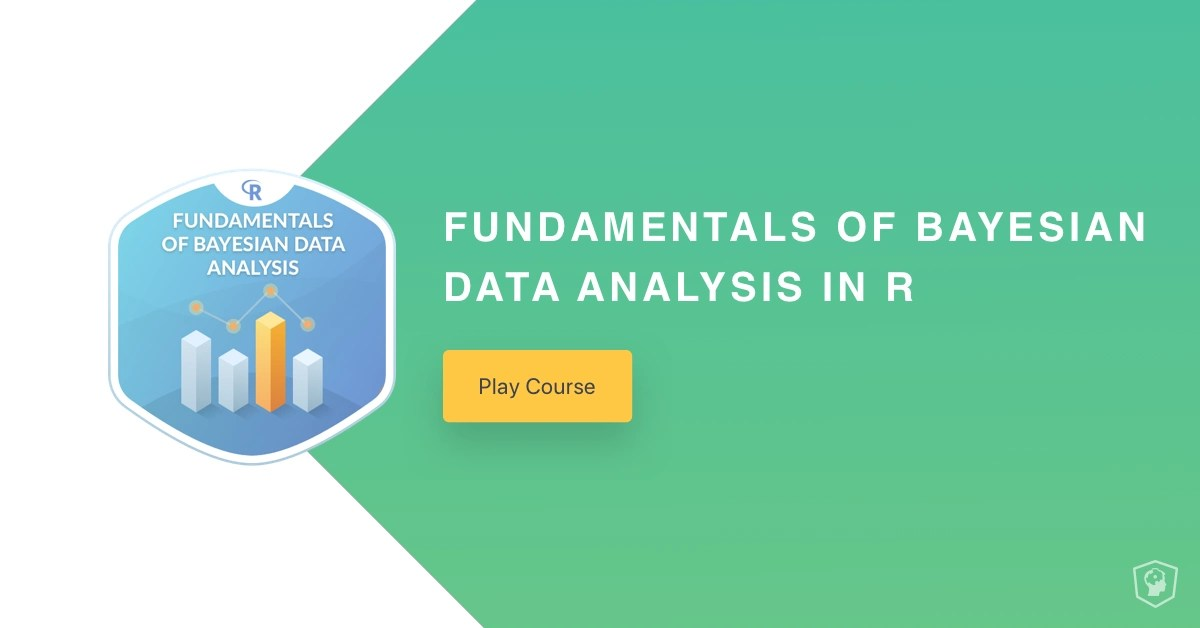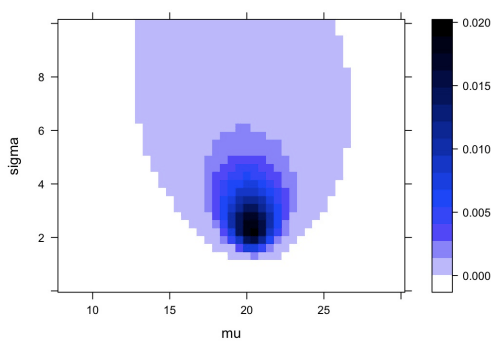Want to share your content on R-bloggers? click here if you have a blog, or here if you don't.### Course Description

Bayesian data analysis is an approach to statistical modeling and machine learning that is becoming more and more popular. It provides a uniform framework to build problem specific models that can be used for both statistical inference and for prediction. This course will introduce you to Bayesian data analysis: What it is, how it works, and why it is a useful tool to have in your data science toolbox.

### Chapter 1: What is Bayesian Data Analysis? (Free)

This chapter will introduce you to Bayesian data analysis and give you a feel for how it works.### Chapter 2: How does Bayesian inference work?

In this chapter we will take a detailed look at the foundations of Bayesian inference.### Chapter 3: Why use Bayesian Data Analysis?

This chapter will show you four reasons why Bayesian data analysis is a useful tool to have in your data science tool belt.### Chapter 4: Bayesian inference with Bayes’ theorem

Learn what Bayes theorem is all about and how to use it for statistical inference.### Chapter 5: More parameters, more data, and more Bayes

Learn about using the Normal distribution to analyze continuous data and try out a tool for practical Bayesian analysis in R.